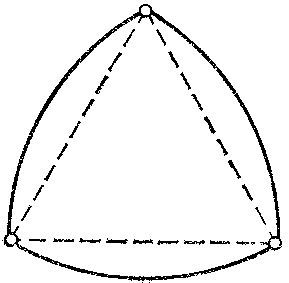Tidbit 34: Do Non-Circular Rollers Exist?

Prepared by:

Joseph Malkevitch
Mathematics and Computing Department
York College (CUNY)
Jamaica, NY 11451

email:

malkevitch@york.cuny.edu

web page:

http://york.cuny.edu/~malk/

Imagine that you are planning to move a flat bottomed cart along the ground on a set of rollers, so that the cart moves in a level manner. (Think of this problem in two dimensions - not too realistic but we have to start somewhere!)

Surely the only choice for the rollers must be circles of equal radius. Think again! It turns out there are infinitely many choices for such rollers. Some of the easier ones to describe have corner points and are not smooth, but there are, in fact, infinitely many smooth choices for rollers.

We will restrict our attention to rollers that are convex, that is, sets with the property that given any two points in the set, the line segment joining these points is also in the set. (Without this condition one could use as rollers circles with other shapes carved out from within the circles. Our question is not a trick one in this particular way.)

Convex sets have many remarkable properties that make it quite fascinating to study them. To avoid problems that come from topological issues we will assume that the convex sets we discuss contain all their boundary points. Thus we will not look at squares or circles with their boundary points thrown away.

If you were unable to meet the challenge of finding a non-circular roller
you have good company. Most people have trouble finding an example that works, though once one sees the solution, like so many other problems in mathematics that are hard to answer on one's own, the solution is charmingly simple.

Here is the simplest solution:

Start with an equilateral triangle, of side length a. Say its vertices are A, B, and C. Putting a compass at draw a circular arc from B to C with radius A. Repeat this procedure for the other two vertices of the triangle. The result is a set R bounded by there equal length circular arcs. Now imagine that one takes a vise with parallel jaws set at a distance apart of more than a, and one slowly closes the vise until it just grips the shape we just created. The distance between the jaws will be exactly a, and this will be true regardless of the position we initially place our set R in.The construction above yields a Reuleaux Triangle, named for the French engineer who first discovered it. One can generalize the construction above to obtain the Reuleaux polygons, starting with any odd sided regular polygon. Sets of this type are often called sets of constant breadth or constant width.

In order to construct a smooth example of a set of constant breadth on can extend each of the sides of the equilateral triangle beyond the vertices the same amount h, say small compared with the side length, beyond the vertices. Using arcs of circles of two different radii, one for the the small extensions from the vertices of the triangle and the other as in the construction above.

References:

Wells, D., The Penguin Dictionary of Curious and Interesting Geometry, Penguin, London, 1991.

Back to list of tidbits